# 英雄哪里出来

## Asia Hefei Online 2008 解题报告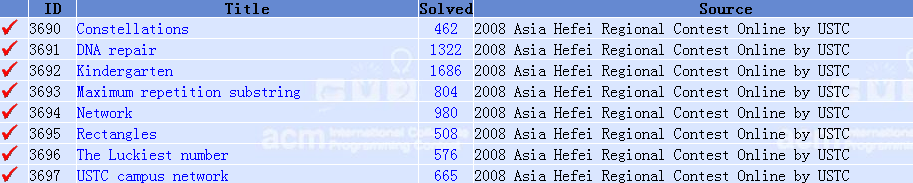A. Constellations

PKU 3690 http://poj.org/problem?id=3690

KMP相关算法可以参阅：

http://www.cppblog.com/menjitianya/archive/2014/06/20/207354.html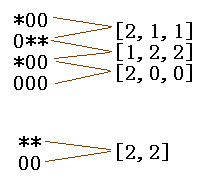1 ‘*’代表二进制的1, ’0’代表二进制的0

B. DNA repair

PKU 3691 http://poj.org/problem?id=3691

题意：给定N(N <= 50)个长度不超过20的模式串，再给定一个长度为M(M <= 1000)的目标串S，求在目标串S上最少改变多少字符，可以使得它不包含任何的模式串（所有串只有ACGT四种字符）。

题解：AC自动机 + 动态规划

Node{

Node *next;   // 能够到达的四个状态 的结点指针

int  id;         // 状态ID，用于到数组下标的映射

int  val;        // 当前状态是否是一个非法状态 （以某些模式串结尾）

}

DP[i][j]表示长度为i (i <= 1000)，状态为j(j <= 50*20 + 1)的字符串变成目标串S需要改变的最少字符，设初始状态j = 0，那么DP = 0，其他均为无穷大。从长度ii+1进行状态转移，每次转移枚举共四个字符(ACGT)，如果枚举到的字符和S对应位置相同则改变值T=1，否则T=0；那么有状态转移方程 DP[i][j] = Min{ DP[i-1][ fromstate ] + T, fromstate为所有能够到达j的状态 };最后DP[n][j]中的最小值就是答案。

C. Kindergarten

PKU 3692 http://poj.org/problem?id=3692

题意：给定G(G <= 200)个女孩和B(B <= 200)个男孩，以及M(0 <= M <= G*B)条记录(x, y)表示x号女孩和y号男孩互相认识。并且所有的女孩互相认识，所有的男孩互相认识，求找到最大的一个集合使得所有人都认识。

D. Maximum repetition substring

PKU 3693 http://poj.org/problem?id=3693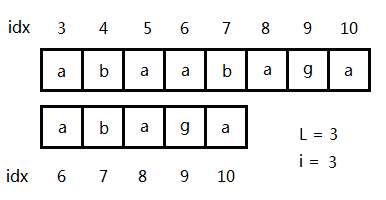2

1) 枚举重复子串的长度L，初始化new_flag标记为true

2) 枚举i，计算S[i*L ... N]S[(i+1)*L ... N]的最长公共前缀T

a) 如果T < Lnew_flag标记为true

b) 如果T >= L，判断new_flag是不是为false，如果为false，说明以S[i*L]为首的串和S[(i-1)*L]为首的串的最长公共前缀大于等于T，跳转到2)；否则转3)

3) 因为S[i*L, (i+1)*L]有重复子串，但是字典序不一定最小，所以还需要枚举区间 [i-L+1, i+L]，看是否存在字典序更小的子串，比较字典序这一步可以直接使用后缀数组计算出来的rank值进行比较。

RMQ相关算法可以参阅：

E. Network

PKU 3694 http://poj.org/problem?id=3694

题解：无向图割边、最近公共祖先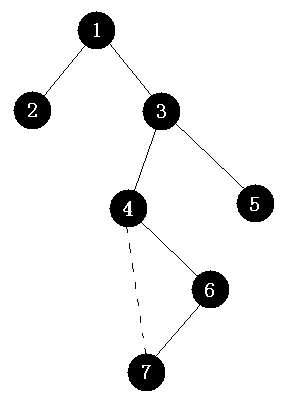3

如图3，图中实线表示树边，虚线表示原图中的边，但是进行tarjan计算的时候7这个结点被(6, 7)这条边“捷足先登”了，于是(4, 7)成为了一条冗余边，计算完后这个图的割边为(1, 2)(1,3)(3, 4)(3, 5)，分别标记bridgebridgebridgebridgetrue

F. Rectangles

PKU 3695 http://poj.org/problem?id=3695

题意：给定N(N <= 20)个矩形，以及M(M <= 105)次询问，询问R(R <= N)个矩形的并。

题解：离散化 + 暴力( 容斥原理 )

离散化：由于矩形很少，所以可以将它们的XY坐标分别离散到整点，两个维度分别离散，点的总数不会超过2N，对于本次询问，利用前一次询问的结果进行面积的增减，对每个矩形进行判断，一共有两种情况：

1）这个矩形前一次询问出现，本次询问不出现，对它的所有离散块进行自减操作，如果某个离散块计数减为0，则总面积减去这个离散块的面积；

2）这个矩形前一次询问没出现，本次询问出现，对它的所有离散块进行自增操作，如果某个离散块计数累加后为1，则总面积加上这个离散块的面积；

容斥原理：对于每个询问，利用dfs枚举每个矩形取或不取，取出来的所有矩形作相交操作，所有[奇数个矩形交]的面积和所有[偶数个矩形交]的面积和 就是答案，因为是dfs枚举，所以在枚举到某次相交矩形面积为0的时候就不需要再枚举下去了，算是一个比较强的剪枝。

如图4，红色区域为被覆盖了一次的区域，橙色区域为被覆盖了两次的区域，黄色区域为被覆盖了三次的区域，那么先将所有的三个矩形加起来，然后需要减掉重叠的部分，重叠的减掉后发现，重叠的部分多减了，即图中黄色的部分被多减了一次，需要加回来。所以容斥原理可以概括为：奇数加，偶数减。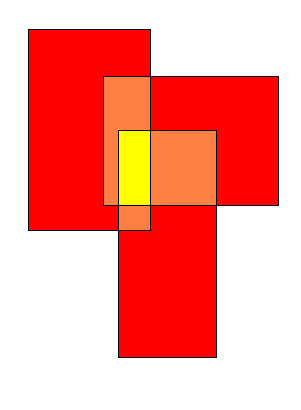4

G. The Luckiest number

PKU 3696 http://poj.org/problem?id=3696

8*(10N-1)/9 = KL     (其中K为任意正整数)  (1)

(10N-1) = 9KL/8                            (2)

A = L/GCD(8, L),  B = 8/GCD(8, L)

(10N-1) = 9K*A / B                          (3)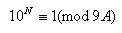(4)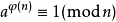5

(ψ(n)表示n的欧拉函数，即小于等于n并且和n互素的数的个数)

1 #define LL __int64
2
3 //计算 a*b % mod
4 LL Produc_Mod(LL a, LL b, LL mod) {
5        LL sum = 0;
6        while(b) {
7               if(b & 1) sum = (sum + a) % mod;
8               a = (a + a) % mod;
9               b >>= 1;
10        }
11        return sum;
12 }
13
14
15 //计算a^b % mod
16 LL Power(LL a, LL b, LL mod) {
17        LL sum = 1;
18        while(b) {
19               if(b & 1) sum = Produc_Mod(sum, a, mod);
20               a = Produc_Mod(a, a, mod);
21               b >>= 1;
22        }
23        return sum;
24 }

H. USTC campus network

PKU 3697 http://poj.org/problem?id=3697

posted on 2014-06-28 19:48 英雄哪里出来 阅读(1724) 评论(0)  编辑 收藏 引用 所属分类: 区域赛 解题报告